Ex 5.1

Chapter 5 Class 12 Continuity and Differentiability
Serial order wise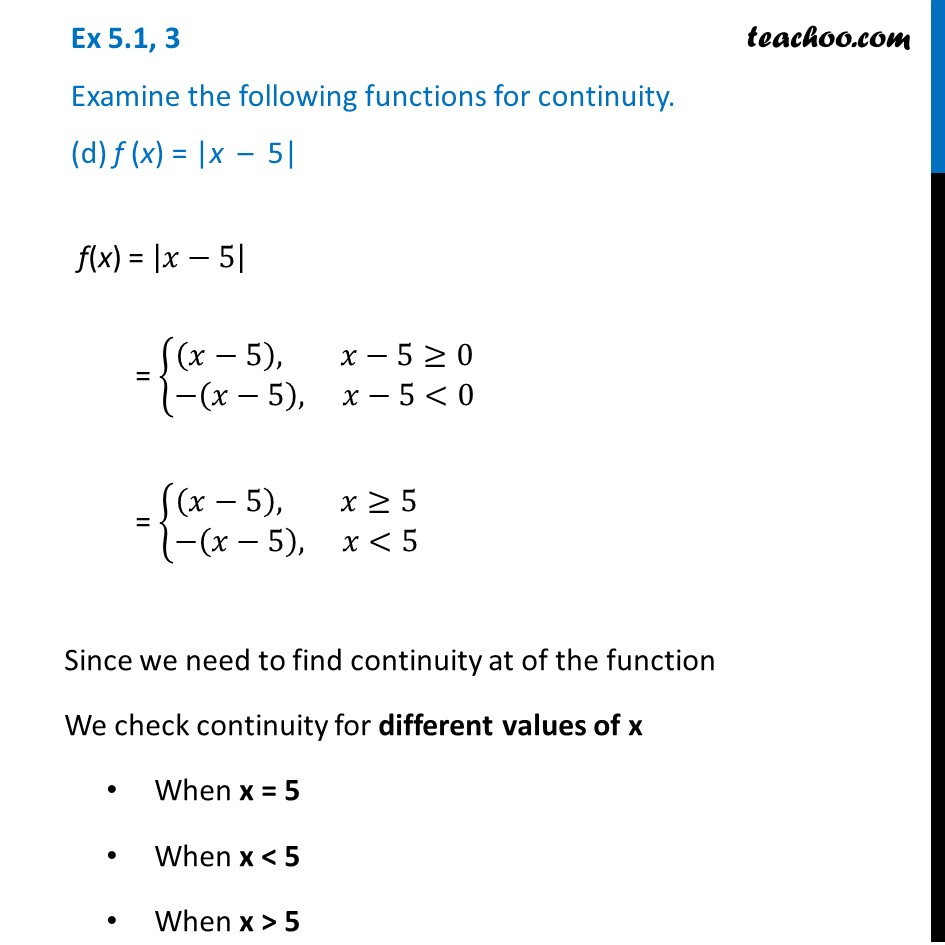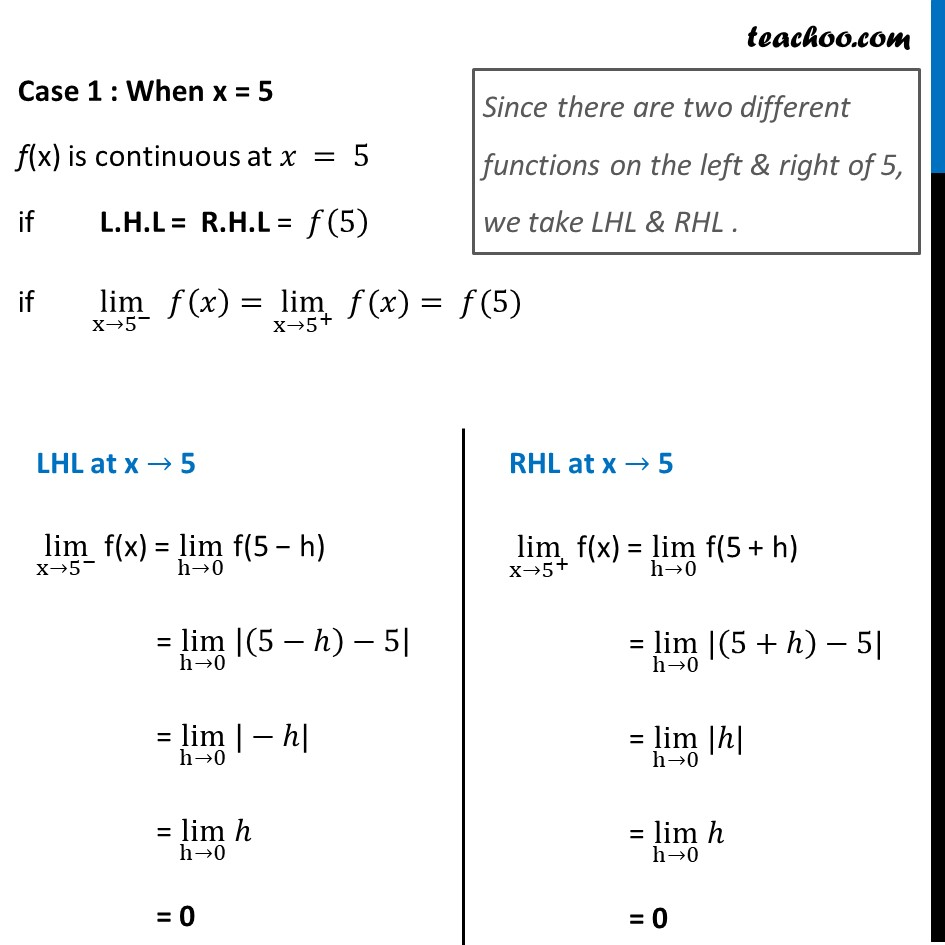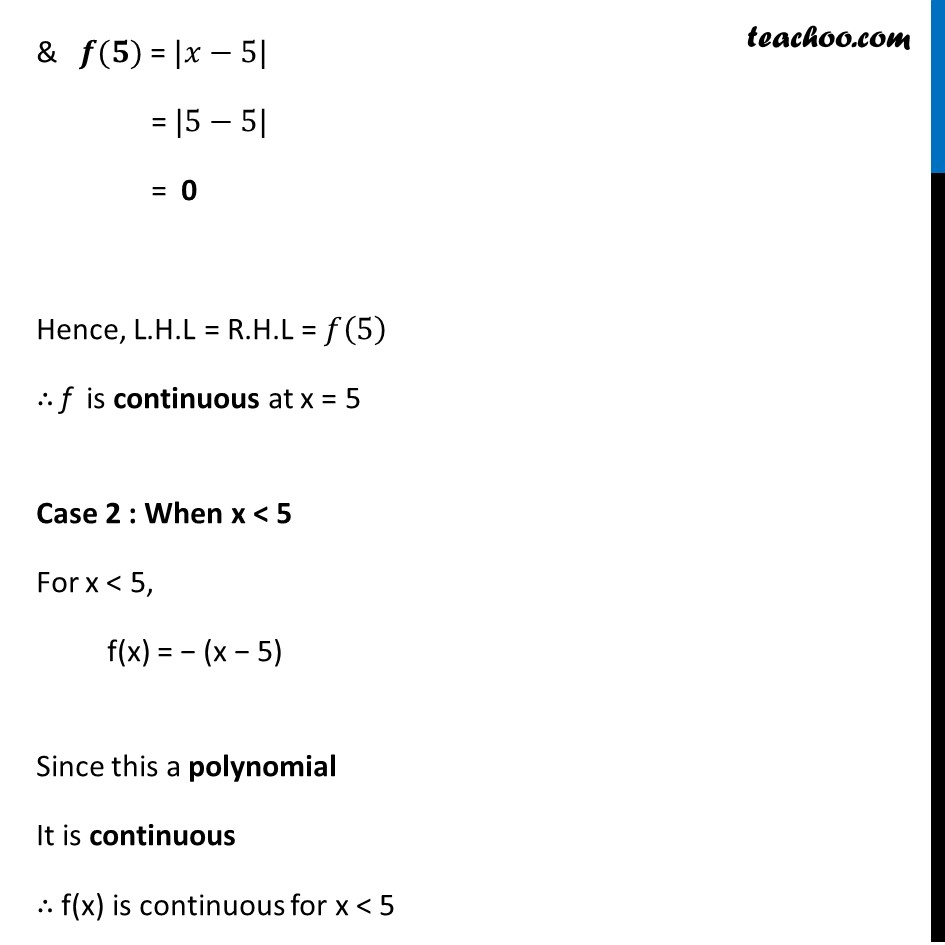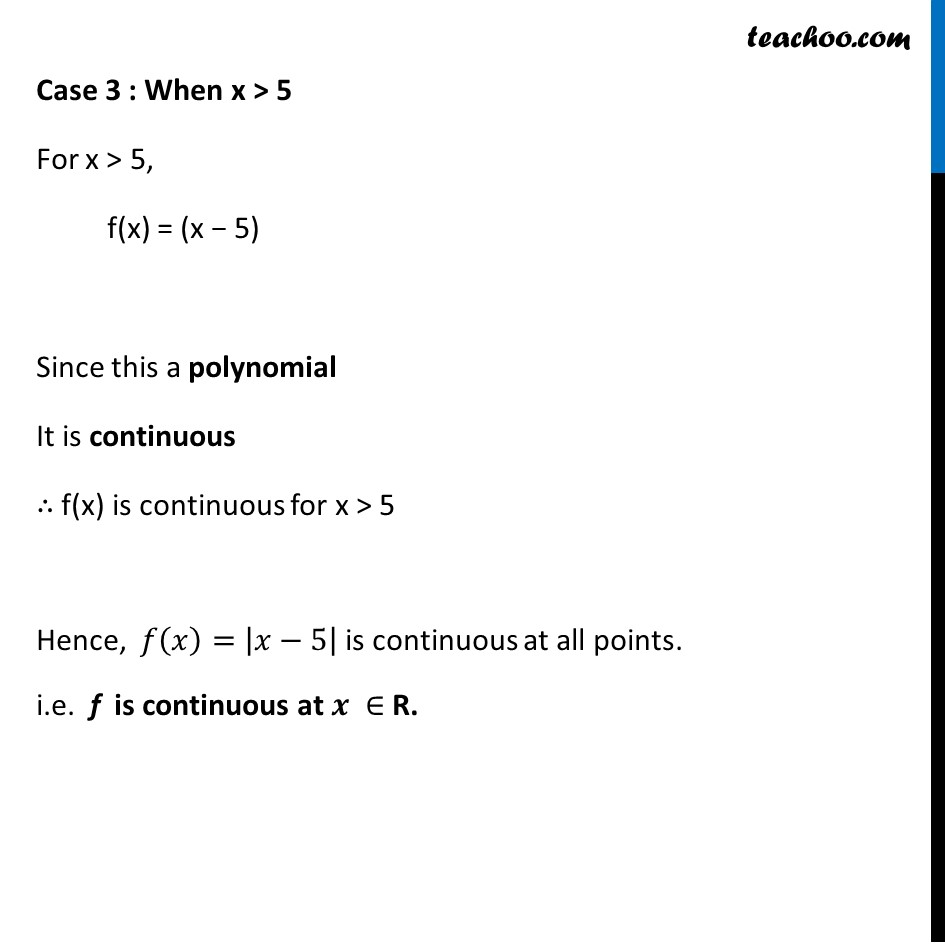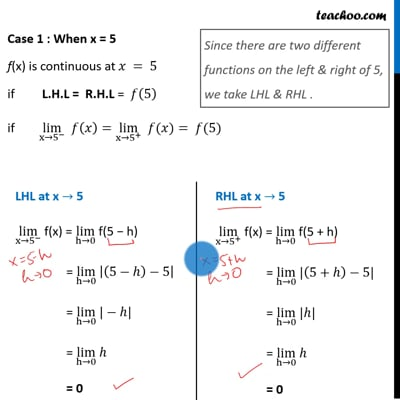This video is only available for Teachoo black users

Introducing your new favourite teacher - Teachoo Black, at only ₹83 per month

### Transcript

Ex 5.1, 3 Examine the following functions for continuity. (d) f (x) = |x – 5| f(x) = |𝑥−5| = {█((𝑥−5), 𝑥−5≥0@−(𝑥−5), 𝑥−5<0)┤ = {█((𝑥−5), 𝑥≥5@−(𝑥−5), 𝑥<5)┤ Since we need to find continuity at of the function We check continuity for different values of x When x = 5 When x < 5 When x > 5 Case 1 : When x = 5 f(x) is continuous at 𝑥 = 5 if L.H.L = R.H.L = 𝑓(5) if lim┬(x→5^− ) 𝑓(𝑥)=lim┬(x→5^+ ) " " 𝑓(𝑥)= 𝑓(5) Since there are two different functions on the left & right of 5, we take LHL & RHL . LHL at x → 5 lim┬(x→5^− ) f(x) = lim┬(h→0) f(5 − h) = lim┬(h→0) |(5−ℎ)−5| = lim┬(h→0) |−ℎ| = lim┬(h→0) ℎ = 0 RHL at x → 5 lim┬(x→5^+ ) f(x) = lim┬(h→0) f(5 + h) = lim┬(h→0) |(5+ℎ)−5| = lim┬(h→0) |ℎ| = lim┬(h→0) ℎ = 0 & 𝒇(𝟓) = |𝑥−5| = |5−5| = 0 Hence, L.H.L = R.H.L = 𝑓(5) ∴ f is continuous at x = 5 Case 2 : When x < 5 For x < 5, f(x) = − (x − 5) Since this a polynomial It is continuous ∴ f(x) is continuous for x < 5 Case 3 : When x > 5 For x > 5, f(x) = (x − 5) Since this a polynomial It is continuous ∴ f(x) is continuous for x > 5 Hence, 𝑓(𝑥)= |𝑥−5| is continuous at all points. i.e. f is continuous at 𝒙 ∈ R.